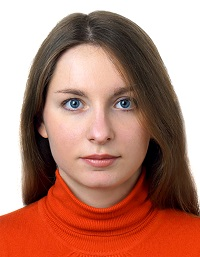## All the truth about reference values

To obtain the exact solution in numerical simulation, the reference values should be set correctly. Find out from this article how to set the reference values and how they affect the accuracy of the solution. We also examined two cases that our users usually face. Forewarned is forearmed.

### Reference, relative and absolute values

In hydro-gasdynamics (in particular, in FlowVision PC), the reference values are ambient temperature and pressure. The temperature and pressure in each cell of the computational domain at each iteration will be calculated in relation to these values — the obtained values will be relative values. By default, the normal atmospheric conditions are set as the reference values: Tref = 273K, pref  = 101000 Pa (1 atm).

If desired, the reference values may always be changed in the Preprocessor -> Region -> General settings.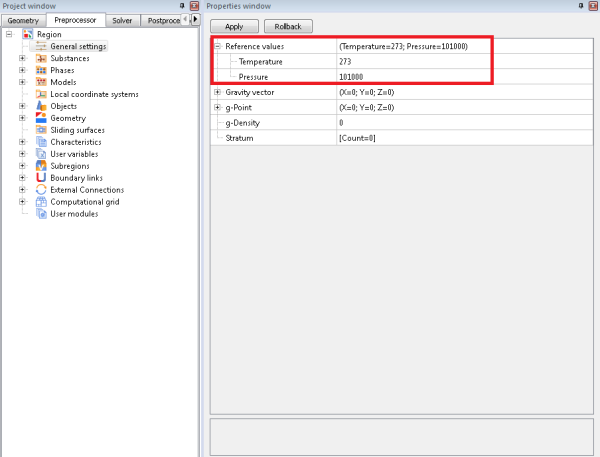To obtain the absolute value of a variable, it is necessary to add a term in the form of a reference value to the relative value:

fa = f + fref,

where fa – absolute value of a variable, f – relative value of a variable, fref – reference value.

### Why should I set the reference values?

Most of the hydro-gas dynamics tasks are solved with respect to environmental parameters: the values of the parameters deviations from those set in the environment are the solution. It is more difficult to compare absolute values of the parameters with each other: the result range increases. For example, to compare the absolute pressures of 101000 Pa and 101385 Pa, it is necessary to work in the range from [0; 101385] Pa. And to compare the same in relative values is much easier - this is a change from 0 to 385 Pa.

### CORRECT setting OF reference VALUES affects THE solution ACCURACY

The differential equations in the FlowVision software code are solved in relative terms. This means that the computations are made regarding deviations of the absolute value on the reference value. In the case when it is possible to calculate this deviation more precisely, the final solution of the equations is more accurate.

If you want to solve a task more precisely, solve it with respect to a certain expected value. The expected values are set as reference and the entire solution will be around them with maximum accuracy.

We want to consider two common situations, that our users face, below.

#### SITUATION 1: HOW TO DETERMINE THE EXPECTED reference VALUES IF THE task IS SOLVED IN A WIDE RANGE OF PARAMETERS?

If the variables of temperature or pressure in the task vary over a wide range, then the deviations from the reference values will be large in any case. But there is nothing to worry about.

Set reference values approximately, at least in terms of order of accuracy.

Also we would like to note that you may always set the initial conditions of the considered physical process, the boundary conditions at the Inlet or Outlet as reference values.

#### SITUATION 2: I WANT TO GET THE CALCULATION RESULTS IN ABSOLUTE VALUES. May I set ZERO refenence values, so the analysis will be IN ABSOLUTE values?

First of all, you can't set the zero reference values - FlowVision will give an error. As a result, users uaually specify not zero, but close to zero (for example, 0.001, etc.) values.

In theory, FlowVision should also calculate with reference values close to zero. However, usually physical processes that we want to simulate do not occur under conditions of absolute zero (0 K) and vacuum (0 Pa). By setting the correct reference values, you provide a solution with acceptable accuracy.

If you want to obtain accurate solution, always set the reference values correctly.

We will describe, how to visualize the results of the solution in absolute values, below.

### How to set project parameters relative to reference values

If you set the expected, non-zero reference values, then all other values of temperature and pressure must be set in relative values: f = fa - fref.
But there are exceptions: we set the temperature and pressure in absolute terms for the substance properties and limiters for calculation.

For clarity, here is a table in which it is indicated where relative and absolute values are set:

 absolute relative Boundary conditions - + Initial conditions - + Modifiers - + Characteristics - + Adaptation(by condition/by solution) - + Building layers - + Substance properties + - Limiters for calculation + -

The temperature and pressure are set in absolute values only in the substance properties and limiters for calculation. In other cases, T and p are set in values relative to the reference ones.

### Absolute values visualization

If you want to display the calculation results in absolute values, use a user variable that contains the formula for converting relative values to absolute: fa = f + fref. The obtained user variable will be visualized in the Postprocessor.

#### EXAMPLE OF ABSOLUTE TEMPERATURE VISUALIZATION

Let's go through the following steps together. As a result, the temperature will be visualized on the layers in PostProcessor in absolute values.

##### step 1

Let's create a local user variable in the Preprocessor: Preprocessor -> Region -> User Variables -> Local.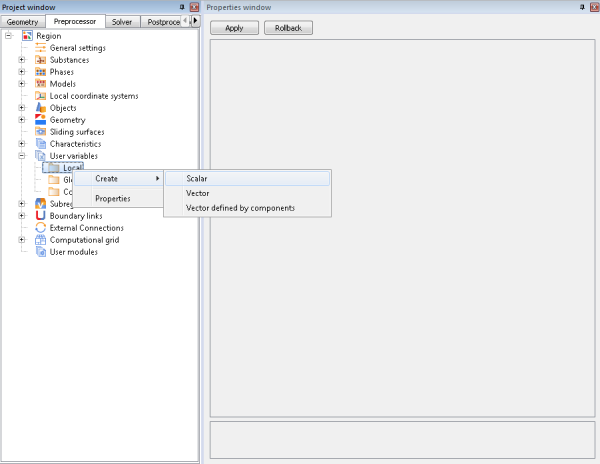##### step 2

Let's write the expression in the formula editor: calculated temperature (physical variables> Phase # > Temperature) + reference temperature (Integral variables> Built-in characteristics> Reference temperature):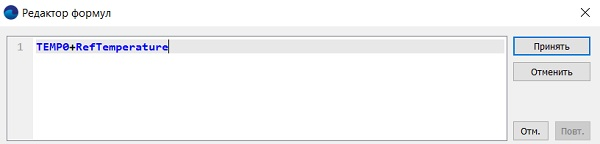##### step 3

Create a layer in the Postprocessor and select the created local variable from the "User Variables" category as a variable.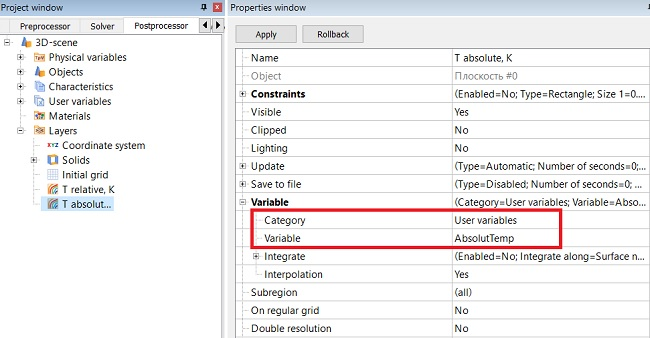Done! The absolute pressure values may be displayed by the same algorithm.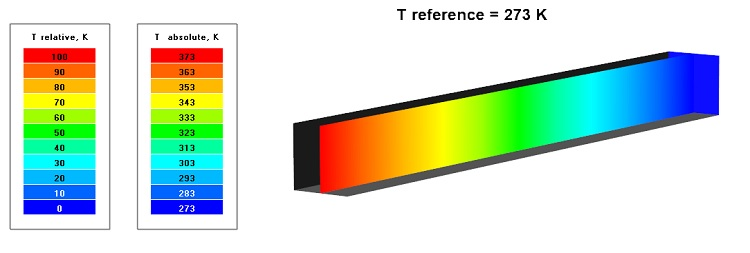Also, information on reference values can be found in the documentation.

### conclusion